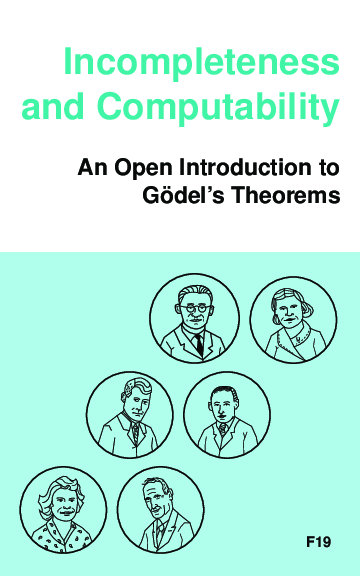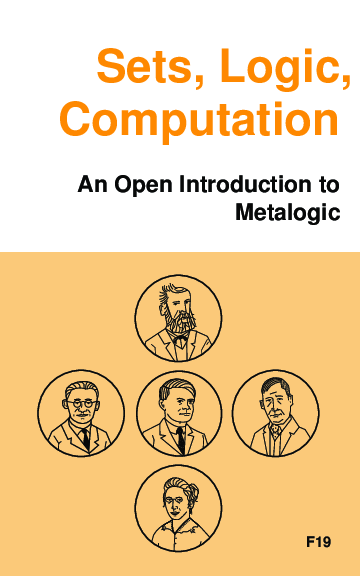# Incompleteness and Computability. An Open Introduction to Gödel’s Theorems

Textbook on Gödel’s incompleteness theorems and computability theory, developed for Calgary’s Logic III course, based on the Open Logic Project. Covers recursive function theory, arithmetization of syntax, the first and second incompleteness theorem, models of arithmetic, second-order logic, and the lambda calculus.# Sets, Logic, Computation. An Open Introduction to Metalogic

Sets, Logic, Computation is an introductory textbook on metalogic. It covers naive set theory, first-order logic, sequent calculus and natural deduction, the completeness, compactness, and Löwenheim-Skolem theorems, Turing machines, and the undecidability of the halting problem and of first-order logic. The audience is undergraduate students with some background in formal logic, e.g., what is covered by forall x.